﻿ 一些涉及黎曼Zeta函数的无穷级数

# 一些涉及黎曼Zeta函数的无穷级数Some Infinite Series Involving the Riemann Zeta Function

Abstract: The series of Riemann functions and the series of hurwitz zeta functions are given by using integral basic identities. The series given is closed. Finally, the numerical series of the zeta function and hurwitz function series are given.

1. 引言与准备

$\zeta \left(s\right)=\left\{\begin{array}{l}\underset{n=1}{\overset{\infty }{\sum }}\frac{1}{{n}^{s}}=\frac{1}{1-{2}^{-s}}\underset{n=1}{\overset{\infty }{\sum }}\frac{1}{{\left(2n-1\right)}^{s}},R\left(s\right)>1\\ \frac{1}{1-{2}^{1-s}}\underset{n=}{\overset{\infty }{\sum }}\frac{{\left(-1\right)}^{n-1}}{{n}^{s}},R\left(s\right)>0,s\ne 1\end{array}$ (1.1)

$\zeta \left(s,a\right)=\underset{n=0}{\overset{\infty }{\sum }}\frac{1}{{\left(n+a\right)}^{s}}$$\mathrm{Re}\left(s\right)>1$$a\ne 0,-1,-2,\cdots$ (1.2)

$\zeta \left(-1\right)=-\frac{1}{12}$$\zeta \left(0\right)=-\frac{1}{2}$${\zeta }^{\prime }\left(0\right)=-\frac{1}{2}\mathrm{ln}\left(2\pi \right)$$\underset{x\to 1}{\mathrm{lim}}\left(\zeta \left(s\right)-\frac{1}{s-1}\right)=\gamma$ (1.3)

$\gamma =\underset{n\to \infty }{\mathrm{lim}}\left(\underset{k=1}{\overset{n}{\sum }}\frac{1}{k}-\mathrm{ln}n\right)\cong 0.577215664901532860606512\cdots$

$\zeta \left(s,a+n\right)=\zeta \left(s,a\right)-\underset{k=0}{\overset{n-1}{\sum }}\frac{1}{{\left(k+a\right)}^{s}}$ (1.5)

$\underset{a}{\overset{b}{\int }}p\left(x\right)\mathrm{cot}x\text{d}x=2\underset{n=1}{\overset{\infty }{\sum }}\underset{a}{\overset{b}{\int }}p\left(x\right)\mathrm{sin}2nx\text{d}x$

$C{l}_{2n}\left(x\right)=\underset{k=1}{\overset{\infty }{\sum }}\frac{\mathrm{sin}\left(kx\right)}{{k}^{2n}}$$n\ge 1$$C{l}_{2n+1}\left(x\right)=\underset{k=1}{\overset{\infty }{\sum }}\frac{\mathrm{cos}\left(kx\right)}{{k}^{2n+1}}$$n\ge 0$

2. 主要结果与证明

1) $\underset{n=1}{\overset{\infty }{\sum }}\frac{\zeta \left(2n\right){x}^{2n-1}}{{\pi }^{2n}}=\frac{1}{2x}-\frac{1}{2}\mathrm{cot}x$$|x|<\pi$ (1)

2) $\underset{n=1}{\overset{\infty }{\sum }}\frac{\zeta \left(2n\right){x}^{2n}}{n{\pi }^{2n}}=\mathrm{ln}x-\mathrm{ln}\mathrm{sin}x$ (2)

3) $\underset{n=1}{\overset{\infty }{\sum }}\frac{\zeta \left(2n\right){x}^{2n+1}}{n\left(2n+1\right){\pi }^{2n}}=-x+x\mathrm{ln}|2\mathrm{sin}x|+\frac{1}{2}C{l}_{2}\left(2x\right)$ (3)

4) $\begin{array}{c}\underset{n=1}{\overset{\infty }{\sum }}\frac{\zeta \left(2n\right){x}^{2n+2}}{n\left(2n+2\right){\pi }^{2n}}=\frac{1}{2}{x}^{2}\mathrm{ln}x-\frac{1}{4}{x}^{2}-\frac{1}{2}{x}^{2}\mathrm{ln}\mathrm{sin}x+{x}^{2}\mathrm{ln}|2\mathrm{sin}x|\\ \text{\hspace{0.17em}}\text{\hspace{0.17em}}+\frac{1}{2}xC{l}_{2}\left(2x\right)+\frac{1}{4}C{l}_{3}\left(2x\right)-\frac{1}{4}\zeta \left(3\right)\end{array}$ (4)

5) $\begin{array}{c}\underset{n=1}{\overset{\infty }{\sum }}\frac{\zeta \left(2n\right){x}^{2n+3}}{n\left(2n+3\right){\pi }^{2n}}=\frac{1}{3}{x}^{3}\mathrm{ln}x-\frac{1}{9}{x}^{3}-\frac{1}{3}{x}^{3}\mathrm{ln}\mathrm{sin}x+\frac{1}{2}{x}^{2}C{l}_{2}\left(2x\right)\\ \text{\hspace{0.17em}}\text{\hspace{0.17em}}-\frac{1}{4}C{l}_{4}\left(2x\right)+\frac{1}{3}{x}^{3}\mathrm{ln}|2\mathrm{sin}x|+\frac{1}{2}C{l}_{3}\left(2x\right)\end{array}$ (5)

6) $\underset{n=1}{\overset{\infty }{\sum }}{\left(-1\right)}^{n-1}\frac{\zeta \left(2n\right){x}^{2n-1}}{{\pi }^{2n}}=-\frac{1}{2x}+\frac{1}{2}\mathrm{coth}x$$|x|\le \pi$ (6)

7) $\underset{n=1}{\overset{\infty }{\sum }}{\left(-1\right)}^{n-1}\frac{\zeta \left(2n\right){x}^{2n}}{n{\pi }^{2n}}=\mathrm{ln}\mathrm{sinh}x-\mathrm{ln}x$$|x|\le \pi$ (7)

2) (1)两端关于x积分，给出(2)式。

3) (2)式两端关于x积分， $\underset{n=1}{\overset{\infty }{\sum }}\frac{\zeta \left(2n\right){x}^{2n+1}}{n\left(2n+1\right){\pi }^{2n}}=x\mathrm{ln}x-x-x\mathrm{ln}x+\underset{0}{\overset{x}{\int }}t\mathrm{cot}t\text{d}t$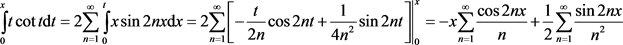4) (2)式两端乘以x再关于x积分

$\underset{n=1}{\overset{\infty }{\sum }}\frac{\zeta \left(2n\right){x}^{2n+2}}{n\left(2n+2\right){\pi }^{2n}}=\frac{1}{2}{x}^{2}\mathrm{ln}x-\frac{1}{4}{x}^{2}-\frac{1}{2}{x}^{2}\mathrm{ln}\mathrm{sin}x+\frac{1}{2}\underset{0}{\overset{x}{\int }}{t}^{2}\mathrm{cot}t\text{d}t$

$\underset{0}{\overset{x}{\int }}{t}^{2}\mathrm{cot}t\text{d}t$ 使用分步积分法，利用积分基本恒等式

$\begin{array}{c}\underset{0}{\overset{x}{\int }}{t}^{2}\mathrm{cot}t\text{d}t=2\underset{n=1}{\overset{\infty }{\sum }}\underset{0}{\overset{t}{\int }}{x}^{2}\mathrm{sin}2nx\text{d}x={2\underset{n=1}{\overset{\infty }{\sum }}\left(-\frac{{t}^{2}}{2n}\mathrm{cos}2t+\frac{t}{2{n}^{2}}\mathrm{sin}2t+\frac{1}{4}\frac{\mathrm{cos}2nt}{{n}^{3}}\right)|}_{0}^{x}\\ =-{x}^{2}\underset{n=1}{\overset{\infty }{\sum }}\frac{\mathrm{cos}2nx}{n}+x\underset{n=1}{\overset{\infty }{\sum }}\frac{\mathrm{sin}2nx}{{n}^{2}}+\frac{1}{2}\underset{n=1}{\overset{\infty }{\sum }}\frac{\mathrm{cos}2nx}{{n}^{3}}-\frac{1}{2}\underset{n=1}{\overset{\infty }{\sum }}\frac{1}{{n}^{3}}\\ ={x}^{2}\mathrm{ln}|2\mathrm{sin}x|+xC{l}_{2}\left(2x\right)+\frac{1}{2}C{l}_{3}\left(2x\right)-\frac{1}{2}\zeta \left(3\right)\end{array}$

5) (2) 式两端乘以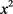，再关于x积分

$\underset{n=1}{\overset{\infty }{\sum }}\frac{\zeta \left(2n\right){x}^{2n+3}}{n\left(2n+3\right){\pi }^{2n}}=\underset{0}{\overset{x}{\int }}\left({t}^{2}\mathrm{ln}t-{t}^{2}\mathrm{ln}\mathrm{sin}t\right)\text{d}t=\frac{1}{3}{x}^{3}\mathrm{ln}x-\frac{1}{9}{x}^{3}-\frac{1}{3}{x}^{3}\mathrm{ln}\mathrm{sin}x+\frac{1}{3}\underset{0}{\overset{x}{\int }}{t}^{3}\mathrm{cot}t\text{d}t$

$\begin{array}{c}\underset{0}{\overset{x}{\int }}{t}^{3}\mathrm{cot}t\text{d}t=2\underset{n=1}{\overset{\infty }{\sum }}\underset{0}{\overset{x}{\int }}{t}^{3}\mathrm{sin}2nt\text{d}t\\ ={\frac{3}{2}{t}^{2}\underset{n=1}{\overset{\infty }{\sum }}\frac{\mathrm{sin}2nt}{{n}^{2}}-\frac{3}{4}\underset{n=1}{\overset{\infty }{\sum }}\frac{\mathrm{sin}2nt}{{n}^{4}}-{t}^{3}\underset{n=1}{\overset{\infty }{\sum }}\frac{\mathrm{cos}2nt}{n}+\frac{3}{2}t\underset{n=1}{\overset{\infty }{\sum }}\frac{\mathrm{cos}2nt}{{n}^{3}}|}_{0}^{x}\\ =\frac{3}{2}{x}^{2}\underset{n=1}{\overset{\infty }{\sum }}\frac{\mathrm{sin}2nx}{{n}^{2}}-\frac{3}{4}\underset{n=1}{\overset{\infty }{\sum }}\frac{\mathrm{sin}2nx}{{n}^{4}}-{x}^{3}\underset{n=1}{\overset{\infty }{\sum }}\frac{\mathrm{cos}2nx}{n}+\frac{3}{2}x\underset{n=1}{\overset{\infty }{\sum }}\frac{\mathrm{cos}2nx}{{n}^{3}}\\ =\frac{3}{2}{x}^{2}C{l}_{2}\left(2x\right)-\frac{3}{4}C{l}_{4}\left(2x\right)+{x}^{3}\mathrm{ln}|2\mathrm{sin}x|+\frac{3}{2}C{l}_{3}\left(2x\right)\end{array}$

6) 根据双曲余切函数定义  $\mathrm{coth}x=\frac{1}{x}+\underset{n=1}{\overset{\infty }{\sum }}\frac{{2}^{2n}{B}_{2n}{x}^{2n-1}}{\left(2n\right)!}$，将贝努里数 ${B}_{2n}$ 代入双曲余切表达式得到(6)式。

7) (6)式两端关于x积分得(7)式。命题1证毕。

1) $\underset{k=0}{\overset{\infty }{\sum }}\frac{{\left(s\right)}_{k}}{k!}\zeta \left(s+k,a\right){t}^{k}=\zeta \left(s,a-t\right)$$\left(|t|<|a|\right)$ (8)

2) $\underset{k=0}{\overset{\infty }{\sum }}\frac{{\left(s\right)}_{k}}{\left(k+1\right)!}\zeta \left(s+k,a\right){t}^{k+1}=\frac{1}{s-1}\zeta \left(s-1,a-t\right)$ (9)

3) $\underset{k=0}{\overset{\infty }{\sum }}\frac{{\left(s\right)}_{k}}{\left(k+2\right)!}\zeta \left(s+k,a\right){t}^{k+2}=\frac{1}{\left(s-1\right)\left(s-2\right)}\zeta \left(s-1,a-t\right)$ (10)

4) $\underset{k=1}{\overset{\infty }{\sum }}\frac{{\left(s\right)}_{k}}{\left(k-1\right)!}\zeta \left(s+k,a\right){t}^{k-1}=\left(s+1\right)\zeta \left(s+1,a-t\right)$ (11)

5) $\underset{k=2}{\overset{\infty }{\sum }}\frac{{\left(s\right)}_{k}}{\left(k-2\right)!}\zeta \left(s+k,a\right){t}^{k-2}=\left(s+1\right)\left(s+2\right)\zeta \left(s+2,a-t\right)$ (12)

$\begin{array}{c}{\left(1+x\right)}^{s}=\underset{k=0}{\overset{\infty }{\sum }}\frac{s\left(s-1\right)\cdots \left(s-k+1\right)}{k!}{x}^{k}\\ =1+\frac{s}{1!}x+\frac{s\left(s-1\right)}{2!}{x}^{2}+\cdots +\frac{s\left(s-1\right)\left(s-2\right)\cdots \left(s-k+1\right)}{k!}{x}^{k}+\cdots \end{array}$

$s<0$ 为负实数，即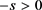，利用升阶乘符号

$\begin{array}{c}{\left(1+x\right)}^{-s}=1+\frac{-s}{1!}x+\frac{-s\left(-s-1\right)}{2!}{x}^{2}+\cdots +\frac{-s\left(-s-1\right)\left(-s-2\right)\cdots \left(-s-k+1\right)}{k!}{x}^{k}+\cdots \\ =1+\frac{-s}{1!}x+\frac{s\left(s+1\right)}{2!}{x}^{2}+\cdots +\frac{{\left(-1\right)}^{k}s\left(s+1\right)\left(s+2\right)\cdots \left(s+k-1\right)}{k!}{x}^{k}+\cdots \\ =\underset{k=0}{\overset{\infty }{\sum }}{\left(-1\right)}^{k}\frac{s\left(s+1\right)\cdots \left(s+k-1\right)}{k!}{x}^{k}=\underset{k=0}{\overset{\infty }{\sum }}{\left(-1\right)}^{k}\frac{{\left(s\right)}_{k}}{k!}{x}^{k}\end{array}$

1) $\begin{array}{c}\zeta \left(s,a-t\right)=\underset{n=0}{\overset{\infty }{\sum }}\frac{1}{{\left(n+a-t\right)}^{s}}=\underset{n=0}{\overset{\infty }{\sum }}\frac{1}{{\left(n+a\right)}^{s}}\frac{{\left(n+a\right)}^{s}}{{\left(n+a-t\right)}^{s}}=\underset{n=0}{\overset{\infty }{\sum }}\frac{1}{{\left(n+a\right)}^{s}}\frac{1}{\frac{{\left(n+a-t\right)}^{s}}{{\left(n+a\right)}^{s}}}\\ =\underset{n=0}{\overset{\infty }{\sum }}\frac{1}{{\left(n+a\right)}^{s}}\frac{1}{{\left(1-\frac{t}{n+a}\right)}^{s}}=\underset{n=0}{\overset{\infty }{\sum }}\frac{1}{{\left(n+a\right)}^{s}}\underset{k=0}{\overset{\infty }{\sum }}\frac{{\left(s\right)}_{k}}{k!}\frac{{t}^{k}}{{\left(n+a\right)}^{k}}\\ =\underset{k=0}{\overset{\infty }{\sum }}\frac{{\left(s\right)}_{k}}{k!}{t}^{k}\underset{n=0}{\overset{\infty }{\sum }}\frac{1}{{\left(n+a\right)}^{s+k}}=\underset{k=0}{\overset{\infty }{\sum }}\frac{{\left(s\right)}_{k}}{k!}\zeta \left(s+k,a\right){t}^{k}\end{array}$ (1.1)成立。

2) 利用赫尔维茨zeta函数积分公式文 ， $\int \zeta \left(s,q\right)\text{d}q=\frac{1}{1-s}\zeta \left(s-1,q\right)$，对(8)式对 $a-t$ 积分1次，2次得到(9)，(10)式。

3) 利用赫尔维茨zeta函数微分公式文 ， $\frac{\text{d}}{\text{d}q}\zeta \left(s-1,q\right)=\left(1-s\right)\zeta \left(s,q\right)$，对(8)式对 $a-t$ 微分1次，2次得到(11)，(12)式。

1)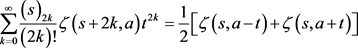(13)

2) $\underset{k=0}{\overset{\infty }{\sum }}\frac{{\left(s\right)}_{2k}}{\left(2k+1\right)!}\zeta \left(s+2k,a\right){t}^{2k+1}=\frac{1}{2}\left[\frac{1}{s-1}\zeta \left(s-1,a-t\right)+\frac{1}{1-s}\zeta \left(s-1,a+t\right)\right]$ (14)

3) $\underset{k=0}{\overset{\infty }{\sum }}\frac{{\left(s\right)}_{2k}}{\left(2k+2\right)!}\zeta \left(s+2k,a\right){t}^{2k+2}=\frac{1}{2}\left[\frac{1}{\left(s-1\right)\left(s-2\right)}\zeta \left(s-2,a-t\right)+\frac{1}{\left(1-s\right)\left(2-s\right)}\zeta \left(s-2,a-t\right)\right]$ (15)

4) $\underset{k=1}{\overset{\infty }{\sum }}\frac{{\left(s\right)}_{2k}}{\left(2k-1\right)!}\zeta \left(s+2k,a\right){t}^{2k-1}=\frac{1}{2}\left[\left(s+1\right)\zeta \left(s+1,a-t\right)+\left(1-s\right)\zeta \left(s+1,a+t\right)\right]$ (16)

5)(17)

$\begin{array}{l}=\frac{1}{2}\left[\underset{n=0}{\overset{\infty }{\sum }}\frac{1}{{\left(n+a\right)}^{s}}\underset{k=0}{\overset{\infty }{\sum }}\frac{{\left(s\right)}_{k}}{k!}\frac{{t}^{k}}{{\left(n+a\right)}^{k}}+\underset{n=0}{\overset{\infty }{\sum }}\frac{1}{{\left(n+a\right)}^{s}}\underset{k=0}{\overset{\infty }{\sum }}\frac{{\left(-1\right)}^{k}{\left(s\right)}_{k}}{k!}\frac{{t}^{k}}{{\left(n+a\right)}^{k}}\right]\\ =\frac{1}{2}\left[\underset{k=0}{\overset{\infty }{\sum }}\frac{{\left(s\right)}_{k}}{k!}{t}^{k}\underset{n=0}{\overset{\infty }{\sum }}\frac{1}{{\left(n+a\right)}^{s+k}}+\underset{k=0}{\overset{\infty }{\sum }}\frac{{\left(-1\right)}^{k}{\left(s\right)}_{k}}{k!}{t}^{k}\underset{n=0}{\overset{\infty }{\sum }}\frac{1}{{\left(n+a\right)}^{s+k}}\right]\\ =\frac{1}{2}\left[\underset{n=0}{\overset{\infty }{\sum }}\frac{2}{{\left(n+a\right)}^{s}}+\frac{2{\left(s\right)}_{2}{t}^{2}}{2!}\underset{n=0}{\overset{\infty }{\sum }}\frac{1}{{\left(n+a\right)}^{s+2}}+\cdots +\frac{2{\left(s\right)}_{2k}{t}^{2k}}{\left(2k\right)!}\underset{n=0}{\overset{\infty }{\sum }}\frac{1}{{\left(n+a\right)}^{s+2k}}+\cdots \right]\\ =\underset{k=0}{\overset{\infty }{\sum }}\frac{{\left(s\right)}_{2k}}{\left(2k\right)!}\zeta \left(s+2k,a\right){t}^{2k}\end{array}$ (13)式成立。

2) 利用赫尔维茨zeta函数积分公式 $\int \zeta \left(s,q\right)\text{d}q=\frac{1}{1-s}\zeta \left(s-1,q\right)$，对(13)式对 $a-t$ 积分1次，2次得到(14)，(15)式。

3) 利用广义黎曼zeta函数微分公式 $\frac{\text{d}}{\text{d}q}\zeta \left(s-1,q\right)=\left(1-s\right)\zeta \left(s,q\right)$，对(13)式对 $a-t$ 微分1次，2次得到(16)，(17)式。

1) $\underset{k=0}{\overset{\infty }{\sum }}\frac{{\left(s\right)}_{2k+1}}{\left(2k+1\right)!}\zeta \left(s+2k,a\right){t}^{2k+1}=\frac{1}{2}\left[\zeta \left(s,a-t\right)-\zeta \left(s,a+t\right)\right]$ (18)

2) $\underset{k=0}{\overset{\infty }{\sum }}\frac{{\left(s\right)}_{2k+1}}{\left(2k+2\right)!}\zeta \left(s+2k,a\right){t}^{2k+2}=\frac{1}{2}\left[\frac{1}{s-1}\zeta \left(s-1,a-t\right)-\frac{1}{1-s}\zeta \left(s-1,a+t\right)\right]$ (19)

3) $\underset{k=0}{\overset{\infty }{\sum }}\frac{{\left(s\right)}_{2k+1}}{\left(2k+3\right)!}\zeta \left(s+2k,a\right){t}^{2k+3}=\frac{1}{2}\left[\frac{1}{\left(s-1\right)\left(s-2\right)}\zeta \left(s-2,a-t\right)-\frac{1}{\left(1-s\right)\left(2-s\right)}\zeta \left(s-2,a-t\right)\right]$ (20)

4) $\underset{k=1}{\overset{\infty }{\sum }}\frac{{\left(s\right)}_{2k+1}}{\left(2k\right)!}\zeta \left(s+2k,a\right){t}^{2k}=\frac{1}{2}\left[\left(s+1\right)\zeta \left(s+1,a-t\right)-\left(1-s\right)\zeta \left(s+1,a+t\right)\right]$ (21)

5) $\underset{k=1}{\overset{\infty }{\sum }}\frac{{\left(s\right)}_{2k+1}}{\left(2k-1\right)!}\zeta \left(s+2k,a\right){t}^{2k-1}=\frac{1}{2}\left[\left(s+1\right)\left(s+2\right)\zeta \left(s+2,a-t\right)-\left(1-s\right)\left(2-s\right)\zeta \left(s+2,a+t\right)\right]$ (22)

3. 一些与黎曼zeta函数相关的数值级数

(1) 一些关于黎曼zeta函数 $\zeta \left(2n\right)$ 的数值级数

1) $\underset{n=1}{\overset{\infty }{\sum }}\frac{\zeta \left(2n\right)}{{2}^{2n-1}\pi }=\frac{1}{\pi }$ ；2) $\underset{n=1}{\overset{\infty }{\sum }}\frac{\zeta \left(2n\right)}{{3}^{2n-1}\pi }=\frac{3}{2\pi }-\frac{1}{2\sqrt{3}}$ ；3) $\underset{n=1}{\overset{\infty }{\sum }}\frac{\zeta \left(2n\right)}{{4}^{2n-1}\pi }=\frac{2}{\pi }-\frac{1}{2}$

1) $\underset{n=1}{\overset{\infty }{\sum }}\frac{\zeta \left(2n\right)}{{2}^{2n}n}=\mathrm{ln}\frac{\pi }{2}$ ；2) $\underset{n=1}{\overset{\infty }{\sum }}\frac{\zeta \left(2n\right)}{{3}^{2n}n}=\mathrm{ln}\frac{\pi }{3}-\mathrm{ln}\frac{\sqrt{3}}{2}$ ；3) $\underset{n=1}{\overset{\infty }{\sum }}\frac{\zeta \left(2n\right)}{{4}^{2n}n}=\mathrm{ln}\frac{\pi }{4}-\mathrm{ln}\frac{\sqrt{2}}{2}$

1) $\underset{n=1}{\overset{\infty }{\sum }}\frac{\zeta \left(2n\right)\pi }{{2}^{2n+1}n\left(2n+1\right)}=-\frac{\pi }{2}+\frac{\pi }{2}\mathrm{ln}2$ ；2) $\underset{n=1}{\overset{\infty }{\sum }}\frac{\zeta \left(2n\right)\pi }{{4}^{2n+1}n\left(2n+1\right)}=-\frac{\pi }{4}+\frac{\pi }{4}\mathrm{ln}\sqrt{2}+\frac{1}{2}G$

3) ${\psi }^{\prime }\left(1/3\right)\underset{n=1}{\overset{\infty }{\sum }}\frac{\zeta \left(2n\right)\pi }{{6}^{2n+1}n\left(2n+1\right)}=-\frac{\pi }{6}+\frac{\sqrt{3}}{12}\left({\psi }^{\prime }\left(1/3\right)-\frac{2}{3}{\pi }^{2}\right)$

1) $\begin{array}{l}\underset{n=1}{\overset{\infty }{\sum }}\frac{\zeta \left(2n\right){\pi }^{2}}{{2}^{2n+3}n\left(n+1\right)}\\ =\frac{1}{8}{\pi }^{2}\mathrm{ln}\frac{\pi }{2}-\frac{{\pi }^{2}}{16}-\frac{{\pi }^{2}}{8}\mathrm{ln}\mathrm{sin}\frac{\pi }{2}+\frac{{\pi }^{2}}{4}\mathrm{ln}2+\frac{\pi }{4}C{l}_{2}\left(\pi \right)+\frac{1}{4}C{l}_{3}\left(\pi \right)-\frac{1}{4}\zeta \left(3\right)\\ =\frac{1}{8}{\pi }^{2}\mathrm{ln}\frac{\pi }{2}-\frac{{\pi }^{2}}{16}-0+\frac{{\pi }^{2}}{4}\mathrm{ln}2+0+\frac{1}{4}\left(-\frac{3}{4}\zeta \left(3\right)\right)-\frac{1}{4}\zeta \left(3\right)\\ =\frac{1}{8}{\pi }^{2}\mathrm{ln}\frac{\pi }{2}-\frac{{\pi }^{2}}{16}+\frac{{\pi }^{2}}{4}\mathrm{ln}2-\frac{7}{16}\zeta \left(3\right)\end{array}$

2) $\begin{array}{l}\underset{n=1}{\overset{\infty }{\sum }}\frac{\zeta \left(2n\right){\pi }^{2}}{{3}^{2n+2}n\left(2n+2\right)}\\ =\frac{1}{18}{\pi }^{2}\mathrm{ln}\frac{\pi }{3}-\frac{{\pi }^{2}}{36}-\frac{{\pi }^{2}}{18}\mathrm{ln}\sqrt{3}+\frac{\pi }{6}C{l}_{2}\left(2\pi /3\right)+\frac{1}{4}C{l}_{3}\left(2\pi /3\right)-\frac{1}{4}\zeta \left(3\right)\\ =\frac{1}{18}{\pi }^{2}\mathrm{ln}\frac{\pi }{3}-\frac{{\pi }^{2}}{36}-\frac{{\pi }^{2}}{36}\mathrm{ln}3+\frac{\pi }{6}\frac{\sqrt{3}}{9}\left[{\psi }^{\prime }\left(1/3\right)-\frac{2}{3}{\pi }^{2}\right]+\frac{1}{4}\left(-\frac{4}{9}\zeta \left(3\right)\right)-\frac{1}{4}\zeta \left(3\right)\\ =\frac{1}{18}{\pi }^{2}\mathrm{ln}\frac{\pi }{3}-\frac{{\pi }^{2}}{36}-\frac{{\pi }^{2}}{36}\mathrm{ln}3+\frac{\pi }{54}\sqrt{3}\left[{\psi }^{\prime }\left(1/3\right)-\frac{2}{3}{\pi }^{2}\right]-\frac{13}{36}\zeta \left(3\right)\end{array}$

3) $\begin{array}{l}\underset{n=1}{\overset{\infty }{\sum }}\frac{\zeta \left(2n\right){\pi }^{2}}{{4}^{2n+2}n\left(2n+2\right)}\\ =\frac{1}{36}{\pi }^{2}\mathrm{ln}\frac{\pi }{4}-\frac{{\pi }^{2}}{64}-\frac{{\pi }^{2}}{32}\mathrm{ln}\frac{\sqrt{2}}{2}+\frac{{\pi }^{2}}{16}\mathrm{ln}\sqrt{2}+\frac{\pi }{8}C{l}_{2}\left(\pi /2\right)+\frac{1}{4}C{l}_{3}\left(\pi /2\right)-\frac{1}{4}\zeta \left(3\right)\\ =\frac{1}{36}{\pi }^{2}\mathrm{ln}\frac{\pi }{4}-\frac{{\pi }^{2}}{64}-\frac{3{\pi }^{2}}{64}\mathrm{ln}2+\frac{\pi }{8}G+\frac{1}{4}\left(-\frac{3}{32}\zeta \left(3\right)\right)-\frac{1}{4}\zeta \left(3\right)\\ =\frac{1}{36}{\pi }^{2}\mathrm{ln}\frac{\pi }{4}-\frac{{\pi }^{2}}{64}-\frac{3{\pi }^{2}}{64}\mathrm{ln}2+\frac{\pi }{8}G-\frac{35}{128}\zeta \left(3\right)\end{array}$

4) $\begin{array}{l}\underset{n=1}{\overset{\infty }{\sum }}\frac{\zeta \left(2n\right){\pi }^{2}}{{6}^{2n+2}n\left(2n+2\right)}\\ =\frac{1}{72}{\pi }^{2}\mathrm{ln}\frac{\pi }{6}-\frac{{\pi }^{2}}{144}-\frac{{\pi }^{2}}{72}\mathrm{ln}\frac{1}{2}+\frac{{\pi }^{2}}{36}\mathrm{ln}\left(2\cdot \frac{1}{2}\right)+\frac{\pi }{12}C{l}_{2}\left(\pi /3\right)+\frac{1}{4}C{l}_{3}\left(\pi /3\right)-\frac{1}{4}\zeta \left(3\right)\\ =\frac{1}{72}{\pi }^{2}\mathrm{ln}\frac{\pi }{6}-\frac{{\pi }^{2}}{144}+\frac{{\pi }^{2}}{72}\mathrm{ln}2+\frac{\pi }{12}\frac{\sqrt{3}}{6}\left[{\psi }^{\prime }\left(1/3\right)-\frac{2}{3}{\pi }^{2}\right]+\frac{1}{4}\left(\frac{1}{3}\zeta \left(3\right)\right)-\frac{1}{4}\zeta \left(3\right)\\ =\frac{1}{72}{\pi }^{2}\mathrm{ln}\frac{\pi }{6}-\frac{{\pi }^{2}}{144}+\frac{{\pi }^{2}}{72}\mathrm{ln}2+\frac{\pi }{72}\sqrt{3}\left[{\psi }^{\prime }\left(1/3\right)-\frac{2}{3}{\pi }^{2}\right]-\frac{1}{6}\zeta \left(3\right)\end{array}$

1) $\begin{array}{l}\underset{n=1}{\overset{\infty }{\sum }}\frac{\zeta \left(2n\right){\pi }^{3}}{{6}^{2n+3}n\left(2n+3\right)}\\ =\frac{1}{648}{\pi }^{3}\mathrm{ln}\frac{\pi }{6}-\frac{{\pi }^{3}}{1944}-\frac{{\pi }^{3}}{648}\mathrm{ln}\frac{1}{2}+\frac{1}{2}\frac{{\pi }^{2}}{36}C{l}_{2}\left(\pi /3\right)-\frac{1}{4}C{l}_{4}\left(\pi /3\right)\text{ }\text{ }+\frac{{\pi }^{3}}{648}\mathrm{ln}1+\frac{1}{2}C{l}_{3}\left(\pi /3\right)\\ =\frac{1}{648}{\pi }^{3}\mathrm{ln}\frac{\pi }{6}-\frac{{\pi }^{3}}{1944}+\frac{{\pi }^{3}}{648}\mathrm{ln}2+\frac{{\pi }^{2}}{72}\frac{\sqrt{3}}{6}\left[{\psi }^{\prime }\left(1/3\right)-\frac{2}{3}{\pi }^{2}\right]\\ \text{\hspace{0.17em}}\text{\hspace{0.17em}}\text{ }\text{ }-\frac{\sqrt{3}}{4}\left\{-\frac{40}{81}\zeta \left(4\right)-\frac{1}{{6}^{4}}\left[\zeta \left(4,\frac{1}{6}\right)+\zeta \left(4,\frac{1}{3}\right)\right]\right\}+\frac{1}{2}\left[\frac{1}{2}\left(1-{2}^{-2}\right)\left(1-{3}^{-2}\right)\zeta \left(3\right)\right]\\ =\frac{1}{648}{\pi }^{3}\mathrm{ln}\frac{\pi }{6}-\frac{{\pi }^{3}}{1944}+\frac{{\pi }^{3}}{648}\mathrm{ln}2+\frac{{\pi }^{2}}{432}\sqrt{3}\left[{\psi }^{\prime }\left(1/3\right)-\frac{2}{3}{\pi }^{2}\right]\\ \text{\hspace{0.17em}}\text{\hspace{0.17em}}\text{ }\text{ }-\frac{\sqrt{3}}{4}\left\{\frac{{3}^{-4}-1}{2}\zeta \left(4\right)-\frac{1}{{6}^{4}}\left[\zeta \left(4,\frac{1}{6}\right)+\zeta \left(4,\frac{1}{3}\right)\right]\right\}+\frac{1}{6}\zeta \left(3\right)\end{array}$

2) $\begin{array}{l}\underset{n=1}{\overset{\infty }{\sum }}\frac{\zeta \left(2n\right){\pi }^{3}}{{4}^{2n+3}n\left(2n+3\right)}\\ =\frac{1}{192}{\pi }^{3}\mathrm{ln}\frac{\pi }{4}-\frac{{\pi }^{3}}{576}-\frac{{\pi }^{3}}{192}\mathrm{ln}\frac{1}{\sqrt{2}}+\frac{{\pi }^{2}}{32}C{l}_{2}\left(\pi /2\right)-\frac{1}{4}C{l}_{4}\left(\pi /2\right)\text{ }\text{ }+\frac{{\pi }^{3}}{192}\mathrm{ln}\sqrt{2}+\frac{1}{2}C{l}_{3}\left(\pi /2\right)\\ =\frac{1}{192}{\pi }^{3}\mathrm{ln}\frac{\pi }{4}-\frac{{\pi }^{3}}{576}+\frac{{\pi }^{3}}{192}\mathrm{ln}2+\frac{{\pi }^{2}}{32}G-\frac{\sqrt{3}}{4}\left[\left({2}^{-4}-1\right)\zeta \left(4\right)+{2}^{1-8}\zeta \left(4,\frac{1}{4}\right)\right]\text{ }\text{ }\\ \text{\hspace{0.17em}}\text{\hspace{0.17em}}+\frac{1}{2}\left[-{2}^{-3}\left(1-{2}^{-2}\right)\zeta \left(3\right)\right]\\ =\frac{1}{192}{\pi }^{3}\mathrm{ln}\frac{\pi }{4}-\frac{{\pi }^{3}}{576}+\frac{{\pi }^{3}}{192}\mathrm{ln}2+\frac{{\pi }^{2}}{32}G\text{ }\text{ }-\frac{\sqrt{3}}{4}\left[-\frac{15}{16}\zeta \left(4\right)+\frac{1}{{2}^{7}}\zeta \left(4,\frac{1}{4}\right)\right]-\frac{3}{64}\zeta \left(3\right)\end{array}$

3) $\begin{array}{l}\underset{n=1}{\overset{\infty }{\sum }}\frac{\zeta \left(2n\right){\pi }^{3}}{{3}^{2n+3}n\left(2n+3\right)}\\ =\frac{1}{81}{\pi }^{3}\mathrm{ln}\frac{\pi }{3}-\frac{{\pi }^{3}}{243}-\frac{{\pi }^{3}}{81}\mathrm{ln}\frac{\sqrt{3}}{2}+\frac{{\pi }^{2}}{18}C{l}_{2}\left(2\pi /3\right)\\ \text{\hspace{0.17em}}\text{\hspace{0.17em}}\text{ }\text{ }-\frac{1}{4}C{l}_{4}\left(2\pi /3\right)+\frac{{\pi }^{3}}{81}\mathrm{ln}\sqrt{3}+\frac{1}{2}C{l}_{3}\left(2\pi /3\right)\\ =\frac{1}{81}{\pi }^{3}\mathrm{ln}\frac{\pi }{3}-\frac{{\pi }^{3}}{243}+\frac{{\pi }^{3}}{81}\mathrm{ln}2+\frac{{\pi }^{2}}{18}\frac{\sqrt{3}}{9}\left[{\psi }^{\prime }\left(1/3\right)-\frac{2}{3}{\pi }^{2}\right]\\ \text{\hspace{0.17em}}\text{\hspace{0.17em}}\text{ }\text{ }-\frac{\sqrt{3}}{4}\left[\frac{{3}^{-4}-1}{2}\zeta \left(4\right)+{3}^{-4}\zeta \left(4,\frac{1}{3}\right)\right]+\frac{1}{2}\frac{1}{2}\left({3}^{1-3}-1\right)\zeta \left(3\right)\\ =\frac{1}{81}{\pi }^{3}\mathrm{ln}\frac{\pi }{3}-\frac{{\pi }^{3}}{243}+\frac{{\pi }^{3}}{81}\mathrm{ln}2+\frac{{\pi }^{2}}{162}\sqrt{3}\left[{\psi }^{\prime }\left(1/3\right)-\frac{2}{3}{\pi }^{2}\right]\\ \text{\hspace{0.17em}}\text{\hspace{0.17em}}\text{ }\text{ }-\frac{\sqrt{3}}{4}\left[-\frac{40}{81}\zeta \left(4\right)+\frac{1}{81}\zeta \left(4,\frac{1}{3}\right)\right]-\frac{2}{9}\zeta \left(3\right)\end{array}$

1) $\underset{n=1}{\overset{\infty }{\sum }}{\left(-1\right)}^{n-1}\frac{2\zeta \left(2n\right)}{{2}^{2n}\pi }=-\frac{1}{\pi }+\frac{1}{2}\mathrm{coth}\frac{\pi }{2}$

2) $\underset{n=1}{\overset{\infty }{\sum }}{\left(-1\right)}^{n-1}\frac{3\zeta \left(2n\right)}{{3}^{2n}\pi }=-\frac{3}{2\pi }+\frac{1}{2}\mathrm{coth}\frac{\pi }{3}$

3) $\underset{n=1}{\overset{\infty }{\sum }}{\left(-1\right)}^{n-1}\frac{\zeta \left(2n\right)}{{2}^{2n}n}=\mathrm{ln}\frac{\pi }{2}+\mathrm{ln}\left(\mathrm{sinh}\frac{\pi }{2}\right)$

4) $\underset{n=1}{\overset{\infty }{\sum }}{\left(-1\right)}^{n-1}\frac{\zeta \left(2n\right)}{{3}^{2n}n}=\mathrm{ln}\frac{\pi }{3}+\mathrm{ln}\left(\mathrm{sinh}\frac{\pi }{3}\right)$

(2) 一些含有升阶乘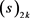的黎曼zeta函数数值级数

1) $\underset{k=0}{\overset{\infty }{\sum }}\frac{{\left(s\right)}_{2k}\zeta \left(s+2k\right)}{{2}^{2k}\left(2k\right)!}=\left({2}^{s}-1\right)\zeta \left(s\right)-{2}^{s-1}$

2) $\underset{k=0}{\overset{\infty }{\sum }}\frac{{\left(s\right)}_{2k}\zeta \left(s+2k\right)}{{3}^{2k}\left(2k\right)!}=\frac{1}{2}\left[\left({3}^{s}-1\right)\zeta \left(s\right)-{3}^{s}\right]$

3)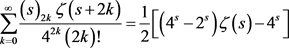4) $\underset{k=0}{\overset{\infty }{\sum }}\frac{{\left(s\right)}_{2k}\zeta \left(s+2k\right)}{{6}^{2k}\left(2k\right)!}=\frac{1}{2}\left[\left({6}^{s}-{3}^{s}-{2}^{s}+1\right)\zeta \left(s\right)-{6}^{s}\right]$

1) 令 $a=1,m=2$

$\begin{array}{l}\underset{k=0}{\overset{\infty }{\sum }}\frac{{\left(s\right)}_{2k}\zeta \left(s+2k\right)}{{2}^{2k}\left(2k\right)!}\\ =\frac{1}{2}\left[\zeta \left(s,1-\frac{1}{2}\right)+\zeta \left(s,1+\frac{1}{2}\right)\right]=\frac{1}{2}\left[\zeta \left(s,\frac{1}{2}\right)+\zeta \left(s,\frac{3}{2}\right)\right]\\ =\frac{1}{2}\left[\zeta \left(s,\frac{1}{2}\right)+\frac{1}{{2}^{s}}+\zeta \left(s,\frac{3}{2}\right)-\frac{1}{{2}^{s}}\right]=\frac{1}{2}\left[\zeta \left(s,\frac{1}{2}\right)+\zeta \left(s,\frac{1}{2}\right)-\frac{1}{{2}^{s}}\right]\\ =\frac{1}{2}\left[2\zeta \left(s,\frac{1}{2}\right)-\frac{1}{{2}^{s}}\right]=\frac{1}{2}\left[2\left({2}^{s}-1\right)\zeta \left(s\right)-\frac{1}{{2}^{s}}\right]=\left({2}^{s}-1\right)\zeta \left(s\right)-{2}^{s-1}\end{array}$

2) 令 $a=1,m=3$

$\begin{array}{l}\underset{k=0}{\overset{\infty }{\sum }}\frac{{\left(s\right)}_{2k}\zeta \left(s+2k\right)}{{3}^{2k}\left(2k\right)!}\\ =\frac{1}{2}\left[\zeta \left(s,\frac{2}{3}\right)+\zeta \left(s,\frac{4}{3}\right)\right]=\frac{1}{2}\left[\zeta \left(s,\frac{2}{3}\right)+\frac{1}{{3}^{s}}+\zeta \left(s,\frac{4}{3}\right)-\frac{1}{{3}^{s}}\right]\\ =\frac{1}{2}\left[\zeta \left(s,\frac{2}{3}\right)+\zeta \left(s,\frac{1}{3}\right)-\frac{1}{{3}^{s}}\right]=\frac{1}{2}\left[\left({3}^{s}-1\right)\zeta \left(s\right)-{3}^{s}\right]\end{array}$

3) 令 $a=1,m=4$

$\begin{array}{l}\underset{k=0}{\overset{\infty }{\sum }}\frac{{\left(s\right)}_{2k}\zeta \left(s+2k\right)}{{4}^{2k}\left(2k\right)!}\\ =\frac{1}{2}\left[\zeta \left(s,\frac{3}{4}\right)+\zeta \left(s,\frac{5}{4}\right)\right]=\frac{1}{2}\left[\zeta \left(s,\frac{3}{4}\right)+\frac{1}{{4}^{s}}+\zeta \left(s,\frac{5}{4}\right)-\frac{1}{{4}^{s}}\right]\\ =\frac{1}{2}\left[\zeta \left(s,\frac{3}{4}\right)+\zeta \left(s,\frac{1}{4}\right)-\frac{1}{{4}^{s}}\right]=\frac{1}{2}\left[\zeta \left(s,\frac{3}{4}\right)+\zeta \left(s,\frac{2}{4}\right)+\zeta \left(s,\frac{1}{4}\right)-\zeta \left(s,\frac{1}{2}\right)-\frac{1}{{4}^{s}}\right]\\ =\frac{1}{2}\left[\left({4}^{s}-1\right)\zeta \left(s\right)-\zeta \left(s,\frac{1}{2}\right)-\frac{1}{{4}^{s}}\right]=\frac{1}{2}\left[\left({4}^{s}-1\right)\zeta \left(s\right)-\left({2}^{s}-1\right)\zeta \left(s\right)-\frac{1}{{4}^{s}}\right]\\ =\frac{1}{2}\left[\left({4}^{s}-{2}^{s}\right)\zeta \left(s\right)-{4}^{s}\right]\end{array}$

4) 令 $a=1,m=6$

$\begin{array}{l}\underset{k=0}{\overset{\infty }{\sum }}\frac{{\left(s\right)}_{2k}\zeta \left(s+2k\right)}{{6}^{2k}\left(2k\right)!}\\ =\frac{1}{2}\left[\zeta \left(s,\frac{5}{6}\right)+\zeta \left(s,\frac{7}{6}\right)\right]=\frac{1}{2}\left[\zeta \left(s,\frac{5}{6}\right)+\frac{1}{{6}^{s}}+\zeta \left(s,\frac{7}{6}\right)-\frac{1}{{6}^{s}}\right]\\ =\frac{1}{2}\left[\zeta \left(s,\frac{5}{6}\right)+\zeta \left(s,\frac{1}{6}\right)-\frac{1}{{6}^{s}}\right]=\frac{1}{2}\left[\zeta \left(s,\frac{5}{6}\right)+\zeta \left(s,\frac{1}{6}\right)-\frac{1}{{6}^{s}}\right]\\ =\frac{1}{2}\left[\zeta \left(s,\frac{5}{6}\right)+\zeta \left(s,\frac{4}{6}\right)+\zeta \left(s,\frac{3}{6}\right)+\zeta \left(s,\frac{2}{6}\right)+\zeta \left(s,\frac{1}{6}\right)\\ \text{\hspace{0.17em}}\text{\hspace{0.17em}}\text{ }\text{ }-\zeta \left(s,\frac{2}{3}\right)-\zeta \left(s,\frac{1}{2}\right)-\zeta \left(s,\frac{1}{3}\right)-\frac{1}{{6}^{s}}\right]\end{array}$

$\begin{array}{l}=\frac{1}{2}\left[\left({6}^{s}-1\right)\zeta \left(s\right)-\zeta \left(s,\frac{1}{3}\right)-\zeta \left(s,\frac{2}{3}\right)-\zeta \left(s,\frac{1}{2}\right)-{6}^{s}\right]\\ =\frac{1}{2}\left[\left({6}^{s}-1\right)\zeta \left(s\right)-\left({3}^{s}-1\right)\zeta \left(s\right)-\left({2}^{s}-1\right)\zeta \left(s\right)-{6}^{s}\right]\\ =\frac{1}{2}\left[\left({6}^{s}-{3}^{s}-{2}^{s}+1\right)\zeta \left(s\right)-{6}^{s}\right]\end{array}$

NOTES

*通讯作者。

 Abramowitz, M. and Stegun, I.A. (Eds.) (1965) Handbook of Mathematical Functions. Dover Publications, New York, 75, 85.

 Whittaker, E.T. and Watson, G.N. (1963) A Course of Modern Analysis: An Introduction to the General Thoery of Infinite Processes and of Analytic Functions; with an Account of the Principal Transcendental Functions. 4th Edition, Cambridge University Press, Cambridge, London, and New York.

 Titchmarsh, E.C. (1951) The Theory of the Riemann Zeta-Function. Oxford University Press (Clarendon), London.

 Connon, D.F. (2007) Some Series and Integrals Involving the Riemann Zetafunction. Binomial Coefficients and the Harmonic Numbers, 5.

 Berndt, B.C. (1985) Ramanujan’s Notbooks, Part 1. Springger-Verlag, 260-263.

 Boros, G. and Moll, V.H. (2004) Irresistible Integrals: Symbolics, Analysis and Experiments in the Evaluation of Integrals. Cambridge University Press.
https://doi.org/10.1017/CBO9780511617041

 Apostol, T.M. (1976) Introduction to Analytic Number Theory. Springer-Verlag, New York, Heidelberg, and Berlin.
https://doi.org/10.1007/978-1-4757-5579-4

Top